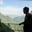Related Tags

fortran
arithmetic
logical
relational
communitycreator

# What are Fortran operators?AKASH BAJWA

An operator is a symbol that explains the compiler to do specific mathematical or logical calculations. We will also discuss operators precedence. Fortran provides the following operator types:

1. Arithmetic operators
2. Logical operators
3. Relational operators

### Arithmetic operators

This table represents all the arithmetic operators that are supported by Fortran. These operators include addition, multiplication, subtraction, division, and more. Assume we have two numbers, X and Y.

Arithmetic operations
 Operator Description Meaning + This Addition operator is used to add two operands Add X with Y - This Subtraction operator is used to subtract second operator from the first one Subtract Y from X * This Multiplication operator is used to multiplies both operands Multiply X with Y / This Division operator is used to divides numerator by denominator Divide X with Y ** This Exponentiation operator is used to raises one operand to the power of the other X with Y as Power(Exponentiation)

### Logical operators

A logical operation includes Boolean values either true (1) or false (0). The basic logical operators are and, or and not. This table represents all the logical operators that are supported by Fortran.

Basic Logical Operators Truth Tables
Logical operations
 Operator Description Demo .AND. It is called AND operator. If both operands are non-zero then returns true Logical conjunction .OR. It is called OR operator to use if any of two operands is not zero then it returns true Logical disjunction .XOR It is called exclusive OR, The negation of OR operation result. Logical exclusive OR .NOT. It is called NOT operator to reverse the logical state of its operand. Logical negation .EQV. It is called EQUIVALENT operator to check equivalence of two operands. Logical equivalence .NEQV. It is called the NON-EQUIVALENT operator to check the non-equivalence of two operands. Logical nonequivalence

### Relational operators

This table represents all the relational operators that are supported by Fortran. These operators help to define multiple operations’ relations between two or more operands. In most cases, relational operators only return 1 or 0, where zero stands for false and one stands for true. Assume X and Y to be two numbers of any type.

Relational operations
 Operator Equivalent Operator Description Meaning != .NE. To check if values of two operands are equal or not. If no then return true X is not equal to Y == .EQ. To check if values of two operands are equal or not. If yes then return true X is Equal to Y > .GT. To check whether the value of left operand is greater than of the right. If yes then returns true X is greater than Y < .LT. To check whether the value of left operand is lesser than of the right. If yes then returns true X is less tah Y <= .LE. To check whether the value of left operand is lesser than or equal to than the right. If yes then return true X is less than or equal to Y >= .GE. To check whether the value of left operand is greater than or equal to than the right. If yes then return true X is greater than or equal to Y

### Fortran: operator precedence

Operator precedence decides the way terms are grouped in an expression and affects how it is evaluated. Some operators have higher precedence than others. For example, multiplication is a higher precedence than addition.

• Example: x = 12 + 5 * 7
• Example: a = 8/2**3

Here, x is assigned 47, not 119 because multiplication has higher precedence than addition. In the second example a is assigned 1 and not 64 because the Exponentiation operator has higher precedence than the Division one.

The following table represents all the operators’ precedence that is supported by Fortran, either from left to right or right to left.

Operator precedence
 Category ﻿﻿﻿﻿Precedence Index Operator Associativity Logical NOT and negative sign 1st .not. (-) Left to right Exponentiation 2nd ** Left to right Multiplicative 3rd */ Left to right Additive 4th +- Left to right Equality 6th == /= Left to right Assignment 9th = Right to Left Logical AND 7th .and. Left to right Logical OR 8th .or. Left to right Relational 5th < <= > >= Left to right

RELATED TAGS

fortran
arithmetic
logical
relational
communitycreator

CONTRIBUTORAKASH BAJWA
RELATED COURSES

View all Courses

Keep Exploring

Learn in-demand tech skills in half the time